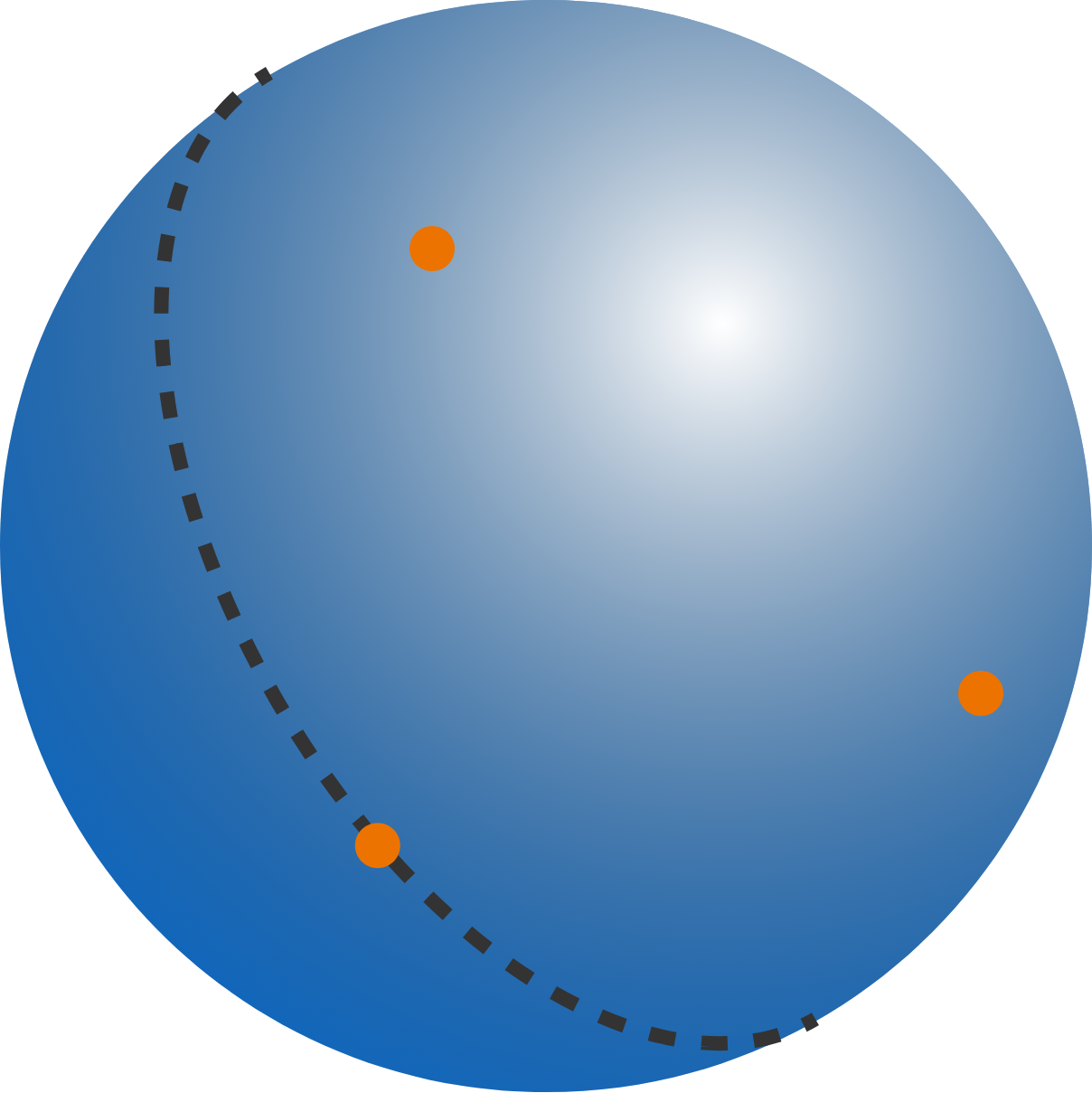# Points on a sphere (part 2)

Geometry Level 2

Suppose someone selects three points at random from the surface of a sphere. Then what is the the probability, in percent, that you can draw a great circle--the largest circle you can possibly draw on the sphere--such that all 3 of the points lie on the same hemisphere (divided by the great circle)?Details and Assumptions:

• Points on the boundary of a hemisphere are considered to lie on the hemisphere.

• Enter, for example, percent like 50% as 50.

×